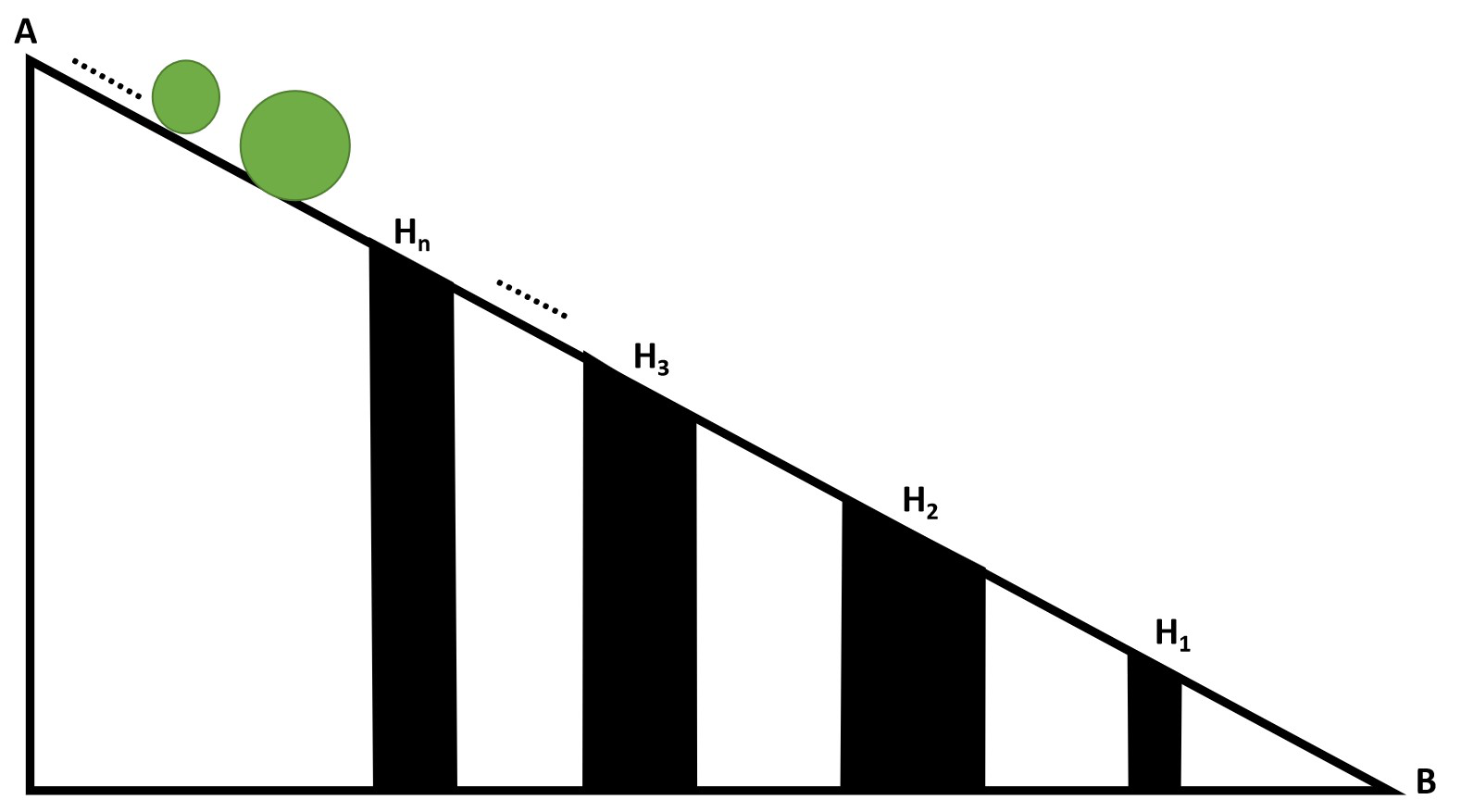Related Articles
TCS Codevita | Holes And Balls
• Difficulty Level : Medium
• Last Updated : 17 Nov, 2020

Given two arrays of H[] and B[] consisting of N and M integers respectively, denoting the diameter of holes and balls respectively. M number of balls are made to roll from A to B on a sloping surface with N holes, each having different depth as shown in the figure below:The task is to find the eventual position of each ball in the order of the ball released considering the following:

• A ball will fall into a hole if its diameter is less than or equal to the diameter of the hole.
• A hole Hi will become full if i numbers of balls fall into it.
• If a hole is full, then no more balls fall into it.
• A ball will reach B from A, if and only if it is not falling into any one of the holes.
• If a ball is in hole Pi, then its position is i. If a ball reached the bottom point B, then take its position as 0.

Examples:

Input: H[] = {21, 3, 6}, B[] = {20, 15, 5, 7, 10, 4, 2, 1, 3, 6, 8}
Output: 1 0 3 0 0 3 3 2 2 0 0
Explanation:
Ball of diameter 20 will fall into the hole H1 and the hole H1 will become full.
Balls with diameter 15, 7 and 10 will reach bottom, since the hole H1 is full and diameters of holes H2 and H3 are less than the diameters of the balls.
Balls with diameters 5, 4 and 2 will fall into the hole H3.
Ball with diameter 1 will fall into the hole H2 since the hole H3 is already full.
Ball with diameter 3 will fall into hole H2.
Balls with diameters 6, and 8 will reach the bottom point B.
The position of ball 20 is 1 because it is in hole H1.
Positions of ball 15, 7, 10, 3, 6, and 8 are 0 because they reached the bottom point B.
Therefore, the balls with diameter 5, 4 and 2 are in the 3rd hole H3, the ball with diameter 1 and 3 are in the 2nd hole H2.

Input: H[] = {20, 15, 10, 5, 25}, B[] = {5, 10, 15, 20, 25, 30, 4, 9, 14, 19}
Output: 5 5 5 5 5 0 4 3 2 1

## Recommended: Please try your approach on {IDE} first, before moving on to the solution.

Approach: Follow the steps below to solve the problem:

• Initialize an array position[] of size N to store the final position of each ball and an array depth[] of size N to store the capacity of each hole.
• Iterate over the range [1,  N] using the variable i and set the initial depth[i] of the hole[i] to i+1.
• Traverse the array ball[] using the variable i and do the following:
• Iterate over the array hole[] using variable j in reverse order.
• Check if the diameter of the hole is greater than or equal to that of the ball, i.e., hole[j] ≥ ball[i], and if that hole is not full, i.e., depth[j] > 0 then, place the ball in that hole by appending j + 1 in the position[] array and decrementing the depth of the hole by 1 and break out of the loop.
• If the ball doesn’t fit in any hole (has reached at end of the slope), then append 0 in the position[] array.
• After the above steps, print the value stored in the array position[] as the result.

Below is the implementation of the above approach:

## Python3

 `# Python program for the above approach`` ` `# Function to find and print the final``# position of balls``def` `ballPositionFinder(diameter_of_holes,``                       ``diameter_of_balls):``   ` `    ``max_hole_limit_counter ``=` `[]``    ``position_value ``=` `[]``     ` `    ``# Stores the positions of balls``    ``ball_positions ``=` `[]`` ` `    ``# Determine the maximum balls a hole``    ``# can store and note the position``    ``# of holes in position_value``    ``for` `i ``in` `range``(``1``, ``len``(diameter_of_holes)``+``1``):``        ``max_hole_limit_counter.append(i)``        ``position_value.append(i)`` ` `    ``# Iterate over all possible holes``    ``# for every ball released``    ``for` `i ``in` `range``(``0``, ``len``(diameter_of_balls)):``        ``for` `j ``in` `range``(``1``, ``len``(diameter_of_holes)``+``1``):`` ` `            ``# Place ball in hole if it fits``            ``# in and if hole is not full``            ``if` `(diameter_of_holes[``-``j] >``=` `diameter_of_balls[i]) ``and` `(``                    ``max_hole_limit_counter[``-``j] !``=` `0``):``               ` `                ``ball_positions.append(position_value[``-``j])``                ``max_hole_limit_counter[``-``j] ``-``=` `1``                ``break`` ` `            ``# If ball has reached at end B``            ``if` `j ``=``=` `len``(diameter_of_holes):``                ``ball_positions.append(``0``)``                ``break`` ` `    ``return` `ball_positions`` ` ` ` `# Driver Code``if` `__name__ ``=``=` `"__main__"``:``   ` `    ``diameter_of_holes ``=` `[``21``, ``3``, ``6``]``     ` `    ``diameter_of_balls ``=` `[``20``, ``15``, ``5``, ``7``, ``10``, ``4``,``                         ``2``, ``1``, ``3``, ``6``, ``8``]`` ` `    ``# Function Call``    ``output ``=` `ballPositionFinder(diameter_of_holes,``                                ``diameter_of_balls)``    ``print``(``*``output, sep ``=``' '``)`
Output:
```1 0 3 0 0 3 3 2 2 0 0
```

Time Complexity: O(N*M)
Auxiliary Space: O(N)

Attention reader! Don’t stop learning now. Get hold of all the important DSA concepts with the DSA Self Paced Course at a student-friendly price and become industry ready.

My Personal Notes arrow_drop_up